# Java IdentityHashMap Tutorial with Examples

#### View more Tutorials: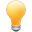Follow us on our fanpages to receive notifications every time there are new articles.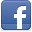Facebook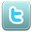Twitter

## 1- IdentityHashMap

IdentityHashMap<K,V> is a class in Java Collection Framework, and implements Map<K,V> interface. IdentityHashMap fully supports all the features specified in Map interface including optional features. Basically IdentityHashMap is quite similar to HashMap, they both use hashing technique to improve data access and storage performance. However, they differ in how data is stored and how keys are compared. IdentityHashMap uses the == operator to compare two keys, while HashMap uses equals method.
``````
public class IdentityHashMap<K,V> extends AbstractMap<K,V>
implements Map<K,V>, java.io.Serializable, Cloneable
``````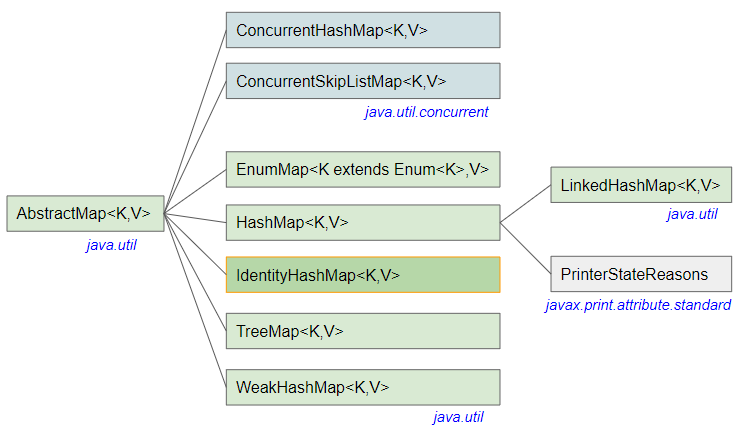IdentityHashMap constructors:
IdentityHashMap() Creates an empty IdentityHashMap object with the default maximum expected size (21). Creates an empty IdentityHashMap object with the specified maximum expected size. Adding more than expectedMaxSize mapping to IdentityHashMap will cause the internal data structure to grow, which can be a bit time consuming. Creates an IdentityHashMap object with mappings copied from a specified Map.

## 2- How does IdentityHashMap work?

Just like HashMap, Java designers used hashing technique for IdentityHashMap class to improve data access and storage performance. Now we will see how this technique is used in IdentityHashMap. Basically, we analyze what happens when we call IdentityHashMap.put(K,V), IdentityHashMap.get(K) and IdentityHashMap.remove(key) methods.
IdentityHashMap manages an array of objects (Object[] table), which can automatically grow in size as needed. And (key,value) pairs are stored at (idx,idx+1) positions.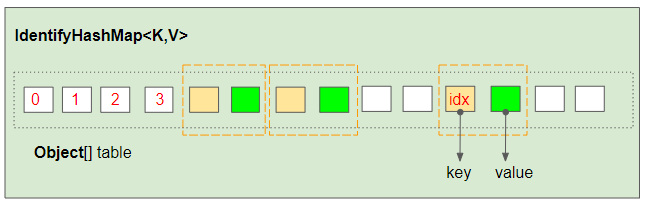The length of internal array is calculated based on the maximum expected size of IdentityHashMap like the example below:
InternalArrayLength_test.java
``````
package org.o7planning.identityhashmap.ex;

public class InternalArrayLength_test {

private static final int MINIMUM_CAPACITY = 4;

private static final int MAXIMUM_CAPACITY = 1 << 29;

private static int capacity(int expectedMaxSize) {
// assert expectedMaxSize >= 0;
return (expectedMaxSize > MAXIMUM_CAPACITY / 3) ? MAXIMUM_CAPACITY
: (expectedMaxSize <= 2 * MINIMUM_CAPACITY / 3) ? MINIMUM_CAPACITY
: Integer.highestOneBit(expectedMaxSize + (expectedMaxSize << 1));
}

public static void main(String[] args) {
for (int i = 1; i < 15; i++) {
int mapSize = i * 25;

int arrayLength = 2 * capacity(mapSize);
System.out.printf("Map size: %3d --> Internal Array length: %d%n",mapSize, arrayLength);
}
}
}
``````
Output:
``````
Map size:  25 --> Internal Array length: 128
Map size:  50 --> Internal Array length: 256
Map size:  75 --> Internal Array length: 256
Map size: 100 --> Internal Array length: 512
Map size: 125 --> Internal Array length: 512
Map size: 150 --> Internal Array length: 512
Map size: 175 --> Internal Array length: 1024
Map size: 200 --> Internal Array length: 1024
Map size: 225 --> Internal Array length: 1024
Map size: 250 --> Internal Array length: 1024
Map size: 275 --> Internal Array length: 1024
Map size: 300 --> Internal Array length: 1024
Map size: 325 --> Internal Array length: 1024
Map size: 350 --> Internal Array length: 2048
``````
IdentityHashMap.put(key,value):
When IdentityHashMap.put(key,value) method is called, IdentityHashMap calculates the expected position to store (notNullKey,value) pair on internal array using the following formula:
``````
private static int hash(Object x, int length) {
int h = System.identityHashCode(x);
// Multiply by -127, and left-shift to use least bit as part of hash
return ((h << 1) - (h << 8)) & (length - 1);
}
// Return default not null Object if key is null.
private static Object maskNull(Object key) {
return (key == null ? NULL_KEY : key);
}

int len = table.length; // Length of Object[] table.
int idx = hash(notNullKey, len);
``````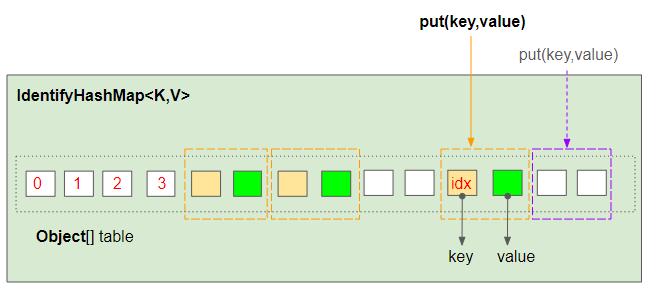(notNullKey,value) pair is expected to be stored at position (idx,idx+1) of the array. (With idx calculated using the above formula).
• If table[idx] is null then (notNullKey,value) pair will be stored at (idx,idx+1) location of the array.
• On the contrary, if table[idx]==notNullKey is true then value will be assigned to table[idx+1].
• Otherwise, the mapping (notNullKey,value) will be stored at (idx+2,idx+3), or (idx+4,idx+5),....
IdentityHashMap.get(key):
When IdentityHashMap.get(key) method is called, IdentityHashMap calculates the expected position found the mapping with notNullKey key on internal array using the same formula:
``````
private static int hash(Object x, int length) {
int h = System.identityHashCode(x);
// Multiply by -127, and left-shift to use least bit as part of hash
return ((h << 1) - (h << 8)) & (length - 1);
}
// Return default not null Object if key is null.
private static Object maskNull(Object key) {
return (key == null ? NULL_KEY : key);
}

int len = table.length; // Length of Object[] table.
int idx = hash(notNullKey, len);
``````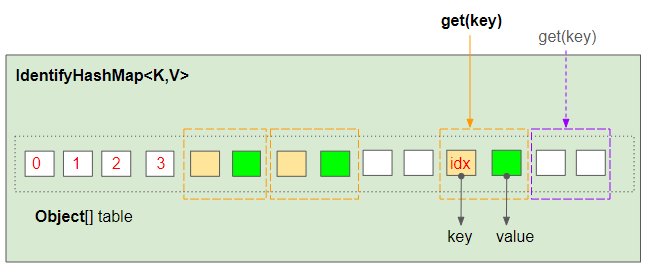Mapping with the notNullKey key is expected to be found at idx index of the array. IdentityHashMap uses the == operator to compare notNullKey and table[idx].
``````
// true or false?
notNullKey == table[idx]
``````
If notNullKey == table[idx] is true then the method will return table[idx+1]. Otherwise, notNullKey will be compared with the next elements at indices idx+2, idx+4,... until a matching index is found or reaches the end of the array. If not found, the method will return null.
IdentityHashMap.remove(key):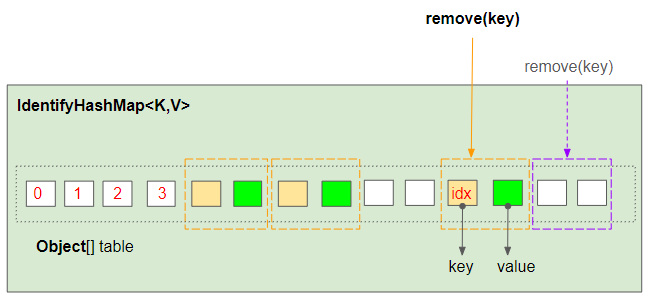IdentityHashMap.remove(key) method works similarly to IdentityHashMap.get(key). If positions (index,index+1) of the mappings to be removed are found, they will be updated to null.
``````
table[index] = null;
table[index+1] = null;
``````
Conclusion:
IdentityHashMap uses System.identityHashCode(key) static method to calculate hashcode of the key. Basically this method returns an integer that is very rarely duplicated. The technique used in IdentityHashMap helps to improve the performance of accessing and storing data. Reduce the use of the == operator to compare objects.
See more about hashing technique used in HashMap:

## 3- Examples

IdentityHashMapEx1.java
``````
package org.o7planning.identityhashmap.ex;

import java.util.IdentityHashMap;

public class IdentityHashMapEx1 {

public static void main(String[] args) {
String key1 = "Tom";
String key2 = new String("Tom");

// key1 == key2 ? false
System.out.println("key1 == key2 ? " + (key1== key2)); // false

IdentityHashMap<String, String> map = new IdentityHashMap<String, String>();

map.put(key1, "Value 1");
map.put(key2, "Value 2");

System.out.println("Map Size: " + map.size());

System.out.println(map);
}
}
``````
Output:
``````
key1 == key2 ? false
Size: 2
{Tom=Value 1, Tom=Value 2}
``````
Basically, all the features of IdentityHashMap conform to Map interface specification, except that it uses == operator to compare keys. This is a slight violation of Map interface specification. (Requires equals method to compare keys).
See more examples:

#### View more Tutorials:

Maybe you are interested

These are online courses outside the o7planning website that we introduced, which may include free or discounted courses.